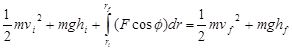$$\require{cancel}$$

# 01. Concepts and Principles

•• Contributed by Paul D'Alessandris
• Professor (Engineering Science and Physics) at Monroe Community College

# The Impulse-Momentum Relation

We've already used the impulse-momentum relation to analyze situations involving constant forces. The relation is typically applied in its component form:Hopefully it's not too much of a stretch to argue that for forces that vary in magnitude or direction that simple summation over a time interval (Dt) must be replaced by an integral over an infinitesimal time (dt):Regardless of whether that forces acting on an object are constant or not, the impulse they exert on the object is precisely equal to the change in the object's momentum.

# The Work-Energy Relation

Our previous encounter with the work-energy relation resulted in:where the forces acting on the object of interest were constant in both magnitude and direction. Again, for forces that vary, I will generalize this result to:where the angle f is the angle between the instantaneous force acting on the object and the instantaneous displacement of the object (dr). Thus, this angle can change as the object moves along its path. Technically, this integral is termed a line integral and its evaluation can be rather complicated.

Also recall from our previous discussion of work-energy that this is not a vector equation, meaning it is not applied independently in each of the coordinate directions.